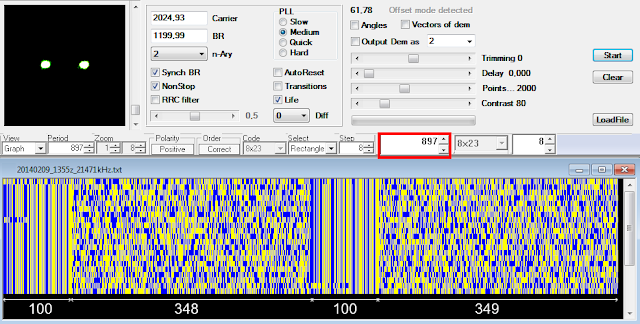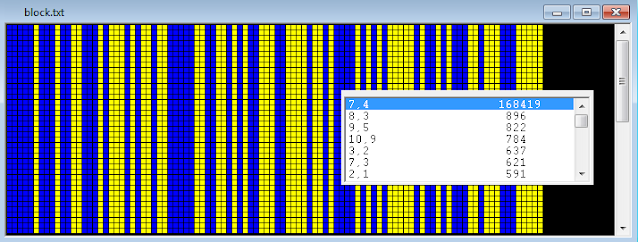## 3 May 2021

### unid, and somewhat peculiar, 1200Bd DBPSK

Cleaning up one of my hard disks I came across an old recording (year 2014) that had no comment file associated with it, so I decided to take a look at it and see what exactly it was. The recording consists of different length bursts, each burst is modulated with PSK2 at a symbol rate of 1200Bd: nothing particularly interesting unless its ACF and, consequently, its period. Indeed, the ACF results in 373.74 ms (Figure 1) that make a length of the period of 448.5 bit (PSK2 @1200Bd), but after the differential decoding the bitstream shows a 897 bit length period, ie just the double of the value obtained using the phase detector.Fig. 1 - 373.74ms ACF corresponding to a 448.5 bit length period

As you know, a such situation is typical in asynchronous framings that have the stop-bit of 1.5 or 2.5 in length: for example, in case of a 5N1.5 framing the bit editor groups two 7.5 bit frames into a single 15 bit pattern because it can't represents a length of half bit. That's just what may happen here: the bit editor reshapes the bistream to a 897 bit length period and draws two frames (Figure 2) consisting of two 100 bit sync sequence, each followed by a data block.Fig. 2 - 897 bit period

However, it's to notice in Figure 2 that the lengths of the two data blocks are different (348 and 349 bit) while the sync sequences have a constant length of 100 bit. Looking at Figure 3, the synchronization patterns are left and right inclined for periods of length 488 and 489 bits respectively: that behavior may confirm the 448.5 bit frame as measured above.Fig. 3 - inclined 100 bit patterns in 448 and 449 bit framings

Talking about it with my friend cryptomaster, we agreed two possible hypothesis:

1) the length of the frame is 897 bit: the framing consists of  two 100 bit sync sequences that are interspersed with two data blocks of  348 and 349 bit; the variable length of the two data blocks and the two sync patterns are a proper feature of this waveform;

2) the length of the frame is 448.5 bit: the framing consists of 100.5 bit for the sync sequence (possible, even if unlikely) followed by 348 bit for the data block.

In order to verify the second assumption,  I set the speed of the PSK demodulator to a double value (2400Bd) so to emerge the missing half bit, if any. The resulting bitstream is shown in Figure 4: the period has the expected length of 1794 bit (2 × 897) and it's possible to see sync sequence patterns of 201 bit in length, ie just the extra bit that was missing.Fig. 4 - 201 bit sequences in the bitstream @2400Bd

Indeed, the 1794 bit period is arranged as: | 201 bit sync  | 696 bit data | 201 bit sync | 696 bit data |;  since the speed of demodulation is doubled, dividing by 2 we get a 448.5 bit frame consisting of 100.5 bit for the sync sequence followed by 348 bit for data:

However, keep in mind that the above bitstream was achieved after a forcing of the demodulation speed to 2400Bd (instead of the effective 1200Bd) and the subsequent differential demodulation: more observations are needed to confirm the 100.5 bit length of the sync sequence.
By the way, the 100 bit sequence may be de-scrambled by the polynomial x^7+x^4+1. Looking for a scrambler polynomial in the 201-bit sequence does not make sense since the way it was obtained.Fig. 5 - x^7+x^4+1 polynomial

Back to the signal, it's interesting to note that some burts have a ~230ms preamble consisting of 8 x PSK2 1200Bd "pulse" (Figure 6): I don't know the reasons and what it can depend on, signal strength and fading seem to indicate that it is not an exchange of messages between two nodes or an ARQ mode.Fig. 6